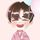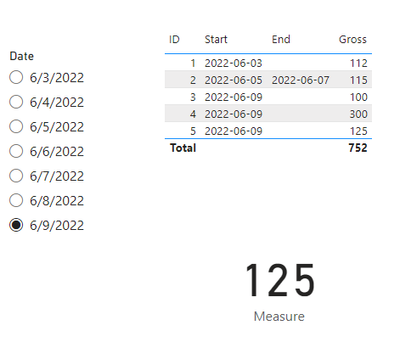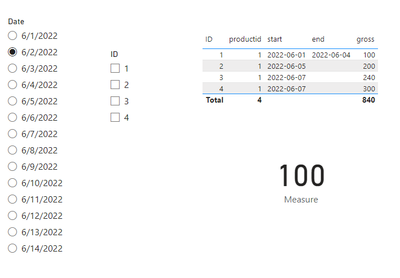cancel
Showing results for
Did you mean:Helper I

## Price calculations help

Hello,

I run into a problem, and i cant find a good solution. I could use some advice 🙂

My problem:

I have a price table that contains gross prices, productid, start date, end date(nullable) . Its a historic table.

ID  productid       start            end        gross
1    1                2022.06.01 2022.06.04    100

2    1                2022.06.05       null          200

3    1                2022.06.07       null          240

4    1                2022.06.07       null          300

i have a date slicer and i need to know the current price based on that slicer. (I have a dedicated measure table, for measures.)

2022.06.02 --> 100

2022.06.05 --> 200

2022.06.07 --> 300 (if it start at the same time table id decide the price)

The SQL:

1 ACCEPTED SOLUTIONCommunity Support

Hi @Akos07 ,

``````Measure =
var _id= CALCULATE(MAX('Table'[ID]),FILTER('Table',[start]<=MAX('Dates'[Date]) && ( [end]=BLANK() || [end]>=MAX('Dates'[Date]))))
return MAXX(FILTER('Table',[ID]=_id),[Gross])``````Best Regards,
Eyelyn Qin
If this post helps, then please consider Accept it as the solution to help the other members find it more quickly.

6 REPLIES 6Community Support

Hi @Akos07 ,

``````Measure =
var _id= CALCULATE(MAX('Table'[ID]),FILTER('Table',[start]<=MAX('Dates'[Date]) && ( [end]=BLANK() || [end]>=MAX('Dates'[Date]))))
return MAXX(FILTER('Table',[ID]=_id),[Gross])``````Best Regards,
Eyelyn Qin
If this post helps, then please consider Accept it as the solution to help the other members find it more quickly.Helper I

Thanks a lot @v-eqin-msft

It was a great help 🙂Community Support

Hi @Akos07 ,

Oh sorry for that.

So if the logic is ——

1.ID is not filtered , use the max gross

2.Select a specific ID, then the specific gross

3.Select multiple IDs, then the sum of gross?

Best Regards,
Eyelyn QinHelper I

ID has no slicer. If you have more records with the same start the hightest ID decide.

1    06.03 112

2    06.05 - 06.07 115
3    06.09 100
4    06.09 300
5    06.09 125

So at 06.03 the price is 112, this is the only record.
06.04 also 112, no changes ID 1 is still valid.
06.05 the price changes to 115. Higher ID.

06.06 same 115

06.07 same 115

06.08 ID 2 is not valid, so the price returns to 112.
06.09 you have 3 records with the same start, but ID 5 is the last record so, the correct price is 125.

(PS: I know its "fantastic" logic 🙂 )Community Support

Hi @Akos07 ,

``Measure = CALCULATE(SUM('Table'[gross]),FILTER('Table',[start]<=MAX('Dates'[Date]) && ( [end]=BLANK() || [end]>=MAX('Dates'[Date])))) ``

Output:Best Regards,
Eyelyn Qin
If this post helps, then please consider Accept it as the solution to help the other members find it more quickly.Helper I

Hey,

Thanks for the reply. I tried someting similar, but the problem is the same. As you can see 06.07 the gross prices adding up 740, it should remain 300.